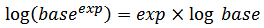# Project Euler – Problem 99 Solution

#### Problem

Comparing two numbers written in index form like 211 and 37 is not difficult, as any calculator would confirm that 211 = 2048 < 37 = 2187.

However, confirming that 632382518061 > 519432525806 would be much more difficult, as both numbers contain over three million digits.

Using base_exp.txt (right click and ‘Save Link/Target As…’), a 22K text file containing one thousand lines with a base/exponent pair on each line, determine which line number has the greatest numerical value.

NOTE: The first two lines in the file represent the numbers in the example given above.

#### Solution

This question looks tricky at first as working out the actual value of each of the base/exponent pair in the file can take a looong time.. but as many others have suggested, you can compare the logarithm of the actual value instead, which can be expressed in the following form:which can be computed very quickly and the rest is easy.